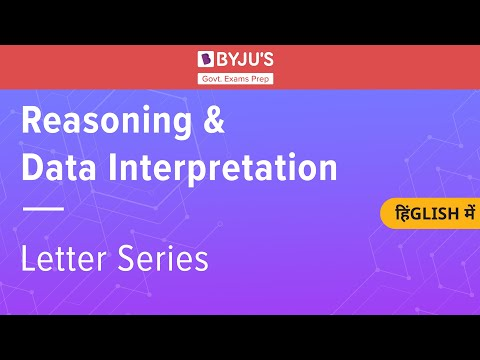# Alphanumeric Series Questions & Answers

Alphanumeric series questions are a combination of numbers, alphabets and symbol-based series which candidates need to answer the questions based on the series.

One of the most frequently asked topics in the various Government exams, the alphanumeric series general cover 2-3 questions in the reasoning ability section.

Along with being easy to answer, the alphanumeric series questions are scoring and one of the most common logical reasoning topic. To check the list of exams in which this section is included, visit the logical reasoning page.

 Ace the Reasoning Ability and General Intelligence Section with the links mentioned below:

In this article, we bring to you 25+ alphanumeric series questions along with their answers. Candidates who are preparing for the upcoming competitive exams must pay emphasis on every topic to crack the examination in the very first attempt.

Given below are a few other logical reasoning concept articles for the candidates o prepare:

## Alphanumeric Series Questions

Given below are alphanumeric series questions, candidates are advised to solve the questions given below and test their preparation level.

And for candidates who are not entirely aware of the concert, can visit the alphanumeric series concept page for a better understanding of the topic.

Directions (Q1 – Q4): Based on the series given below, answer the following questions:

H  #  K  O  &  L  $% X I A V E @ ? D + F M Q 1. How many symbols in the given series are immediately preceded by a vowel? 1. One 2. Two 3. Three 4. Four 5. Five Q 2. Which is the seventh element from the left of the 15th element from left? 1. A 2.$
3. %
4. D
5. ?

Q 3. How many such consonants are there in the given series, each of which is immediately preceded by a vowel and succeeded by a symbol?

1. Four
2. Six
3. None
4. One
5. Two

Q 4. Which element is placed 4th to the right of 11th element from left?

1. D
2. +
3. #
4. ?
5. XDirections (Q5 – Q9): Study the series given below carefully and answer the following questions:

Q  2   K  4  *  $B K + D 5 1 F & R$   O  ^  C

Q 5. If all the symbols in the series are removed, which element will be sixth from the right end of the series?

1. 5
2. R
3. K
4. +
5. B

Q 6. How many symbols are there in between the first vowel from left and the first consonant from the right end of the series?

1. Two
2. Four
3. Three
4. One
5. None of the above

Q 7. How many such symbols are there in the given series which are immediately preceded by a symbol and followed by a consonant?

1. Two
2. Three
3. One
4. None
5. None of the above

Q 8. Which element is second to the right of the fifth element from the left of the series?

1. F
2. K
3. #
4. +
5. B

Q 9. What is the product of all the numbers given in the alphanumeric series?

1. 45
2. 50
3. 40
4. 12
5. 60

Directions (Q10 – Q14): In each of the alphanumeric series given below, find the missing element

Q 10.

A-9  B-16  ?  D-36  E-49

1. C-26
2. C-30
3. C-27
4. C-25
5. C-33

Q 11.

Z-12  X-8  T-0  R-8

1. V-6
2. V-4
3. V-10
4. V-0
5. V-14

Q 12.

P-1  ?  R-27  S-256  T-3125

1. Q-5
2. Q-2
3. Q-6
4. Q-30
5. Q-22

Q 13.

? G-4 H-6 I-8 J-10

1. F-2
2. F-1
3. F-0
4. F-4
5. F-10

Q 14.

A-26  B-25  C-24  X-3  Y-2  ?

1. Z-5
2. Z-22
3. Z-26
4. Z-3
5. Z-1

Candidates can also refer to the links given below:

Directions (Q15 – Q18): Refer to the number given below, and answer the following questions:

234  657  283  647  994

Q 15. If all the digits in each of the given numbers are arranged in ascending order within the number, which will be highest number thus formed?

1. 234
2. 657
3. 283
4. 647
5. 994

Q 16. If the first and last digit of each number is interchanged with each other, which will be the smallest number thus formed?

1. 234
2. 994
3. 657
4. 647
5. 283

Q 17. When all the digits in each number is multiplied with each other, the product of which of these numbers shall be the greatest?

1. 234
2. 657
3. 283
4. 647
5. 994

Q 18. If the digit at one’s place of the largest number is added to the digit at hundreds place of the smallest number. What will be the sum of the two numbers?

1. 12
2. 24
3. 35
4. 23
5. 13

Directions (Q19 – Q22):  Based on the alphanumeric series given below, answer the following questions:

4   G   7   *  $K$   L   ?   #    L   O    @   E    B   +   A   I

Q 19. Which element is placed sixth to the right of the seventh element from left?

1. G
2. #
3. \$
4. @
5. B

Q 20. How many such vowels are there in the given series, each of which is followed by a consonant?

1. Two
2. Three
3. One
4. None
5. None of the above

Q 21. If all the symbols and vowels are eliminated from the series, which element shall be seventh from the right end of the series?

1. 7
2. 4
3. K
4. L
5. B

Q 22. Which element is fourth to the left of the second element from the right end of the series?

1. #
2. *
3. E
4. B
5. @

For all the questions given above, candidates can get the answers at the PDF given below: